#### 期刊菜单

Research on Low Frequency Oscillation Disturbance Source Location of Power System Based on a Prony Method of Simplified Principal Element
DOI: 10.12677/SG.2022.124012, PDF, HTML, XML, 下载: 77  浏览: 113  国家自然科学基金支持

Abstract: Low frequency oscillation occurs frequently in power system. Aiming at the problems of inaccurate positioning of oscillation source and large amount of calculation at present, based on the relationship and characteristics of forced oscillation energy function and energy conversion in the steady-state stage of oscillation, this paper proposes a disturbance source positioning method based on parameter identification on the basis of traditional Prony positioning disturbance source. This method is improved by combining the principal component method, which greatly reduces the amount of calculation and makes the disturbance source positioning more accurate when taking a large amount of data. Finally, a simulation model is established to verify that this method can locate the disturbance source more quickly than the traditional Prony method, and it is easy to perform the calculation online.

1. 引言

2. 基本理论

2.1. Prony算法 

$x\left(n\right)=\underset{i=1}{\overset{p}{\sum }}{b}_{i}{z}_{i}^{n}\left(n=0,\cdots ,N-1\right)$ (1)

$\text{​}{b}_{i}={A}_{i}\mathrm{exp}\left(j{\theta }_{i}\right)$ (2)

${Z}_{i}=\mathrm{exp}\left[\left({\alpha }_{i}+j\omega 2\pi {f}_{i}\right)t\right]$ (3)

$\mathrm{min}z=\underset{n=0}{\overset{N-1}{\sum }}{\left(x\left(n\right)-\stackrel{¯}{x}\left(n\right)\right)}^{2}$ (4)

${A}_{i}=|{b}_{i}|$ (5)

${\theta }_{i}=\mathrm{arctan}\left[\frac{\mathrm{Im}\left({b}_{i}\right)}{\mathrm{Re}\left({z}_{i}\right)}\right]$ (6)

${\alpha }_{i}=\frac{\mathrm{ln}|{Z}_{i}|}{\Delta t}$ (7)

${f}_{i}=\mathrm{arctan}\left[\mathrm{Im}\left({Z}_{i}\right)/\mathrm{Re}\left({Z}_{i}\right)\right]/2\pi \Delta t$ (8)

2.2. Prony算法中的参数选择

Prony算法拟合的结果及精确程度和其参数的选择有紧密的联系，参数选择不当就可能导致Prony算法很有可能无法进行拟合。Prony算法主要参数及和其选择基本标准遵循以下规律。

2.3. 主元法

$\text{ACF}\left(I\right)=E\left[x\left(n\right)x\left(n-1\right)\right]$ (9)

$s\left(f\right)={\sum }_{\text{-}\infty }^{\infty }E\left[x\left(n\right)x\left(n-1\right)\right]{\text{e}}^{-j2\pi kf}$ (10)

$x=\left(\begin{array}{ccc}{p}_{1}\left({f}_{0}\right)& \dots & {p}_{1}\left({f}_{N}\right)\\ ⋮& \ddots & ⋮\\ {p}_{m}\left({f}_{0}\right)& \cdots & {p}_{m}\left({f}_{N}\right)\end{array}\right)$ (11)

$x=T{W}^{\text{T}}=T{W}^{\text{T}}+E\approx \left(\begin{array}{ccc}{t}_{11}& \dots & {t}_{1p}\\ ⋮& \ddots & ⋮\\ {t}_{m1}& \cdots & {t}_{mp}\end{array}\right)$ (12)

${x}_{i}=\left[{t}_{i}{w}_{i},{t}_{i}{w}_{w},\cdots ,{t}_{i}{w}_{n}\right]\approx \left[{t}_{i}{w}_{i},{t}_{i}{w}_{i},\cdots ,{t}_{i}{w}_{p}\right]={t}_{i}\otimes {w}^{\text{T}}$ (13)

2.4. 基于改进的Prony主元方法

$\left\{\begin{array}{l}M\frac{\text{d}\omega }{\text{d}t}=\Delta {p}_{m}-\Delta {p}_{e}-D\Delta \omega \\ \frac{\text{d}\Delta \omega }{\text{d}t}={\omega }_{0}\Delta \omega \end{array}$ (14)

$\int \Delta {p}_{m}\Delta \omega {\omega }_{0}\text{d}t-\int D\Delta \omega {\omega }_{0}\text{d}t$ (15)

$V={V}_{KE}+{V}_{PE}={V}_{M}+{V}_{D}$ (16)

$\Delta {p}_{ij}=\Delta {P}_{mij}\mathrm{cos}\left(\omega t+{\phi }_{1}\right)$ (17)

$\Delta {\omega }_{i}=\Delta {\omega }_{mi}\mathrm{cos}\left(\omega t+{\phi }_{2}\right)$ (18)

$\begin{array}{c}{V}_{pei}=\underset{0}{\overset{t}{\int }}\Delta \mathrm{cos}\left(\omega t+{\phi }_{1}\right)\Delta {\omega }_{mi}\mathrm{cos}\left(\omega t\right)+\frac{1}{4\omega }\Delta {p}_{mij}\Delta {\omega }_{mi}{\omega }_{0}\mathrm{sin}\left(2\omega t+{\phi }_{1}+{\phi }_{2}\right)\\ \text{\hspace{0.17em}}\text{ }+\frac{1}{2}\Delta {p}_{mij}\Delta {\omega }_{mi}{\omega }_{0}\mathrm{cos}\left({\phi }_{1}-{\phi }_{2}\right)+\frac{1}{2}\Delta {p}_{mij}\Delta {\omega }_{mi}{\omega }_{0}t\mathrm{sin}\left({\phi }_{1}+{\phi }_{2}\right)\end{array}$ (19)

$a=\frac{1}{4\omega }\Delta {p}_{mij}\Delta {\omega }_{mi}{\omega }_{0}$ (20)

$b=\frac{1}{2}\Delta {p}_{mij}\Delta {\omega }_{mi}{\omega }_{0}\mathrm{cos}\left({\phi }_{1}-{\phi }_{2}\right)$ (21)

$c=\frac{1}{2}\Delta {p}_{mij}\Delta {\omega }_{mi}{\omega }_{0}t\mathrm{sin}\left({\phi }_{1}+{\phi }_{2}\right)$ (22)

2.5. 流程图分析

1) 步骤1大量电力系统PMU数据，通过Prony构造指数模型建立函数，获得频率、相角等相关信息。

2) 步骤2电力系统频率进行计算自相关函数ACF和功率谱密度S(f)。

3) 步骤3主元法对数据进行降维处理，计算任意两个数据间欧式距离，反映其功率谱相似性。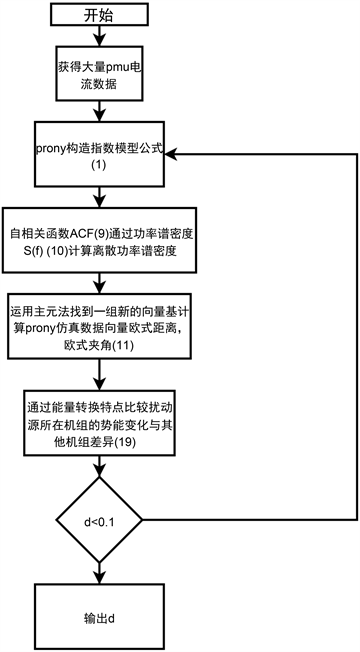Figure 1. Flowchart of Prony combined with principal element method

3. 算例仿真

3.1. Prony参数选择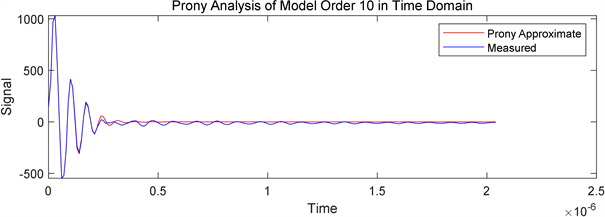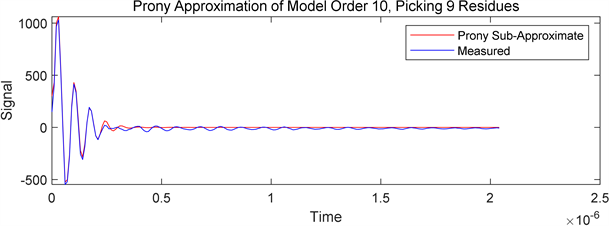Figure 2. Prony analysis results when the sample data are 400 groups and the model order is 10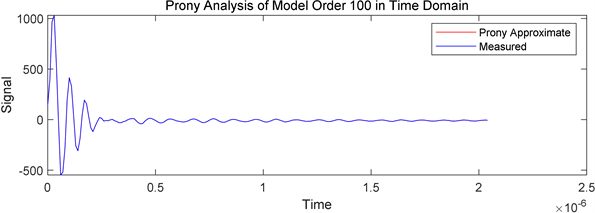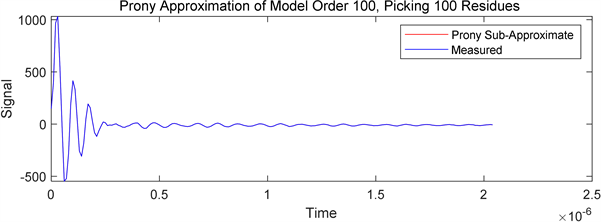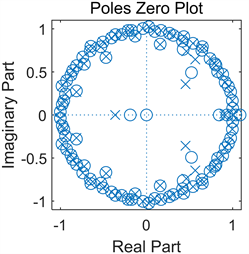Figure 3. Prony analysis results when the sample data are 400 groups and the model order is 100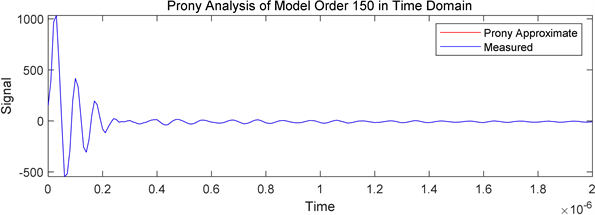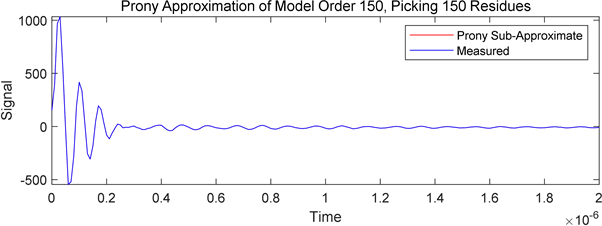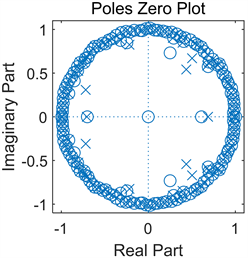Figure 4. Prony analysis results when the sample data are 400 groups and the model order is 150

3.2. Prony结合振荡源定位分析

3.3. Prony定位振荡源分析Table 1. Principal element method iterative oscillation source position data tableFigure 5. Prony analysis results when the sample data are 400 groups and the model order is 150

4. 结论

1) 归纳和分析了不同区域互联电力系统低频振荡的产生机理，并且基于模态和测量数据归纳了一些主要分析方法及低频振荡抑制措施；从理论上进行风电场接入多机互联电网的低频振荡的影响分析。当风电场与电网之间距离、风电场出力等因素变化时，系统总阻尼增量将发生变化，系统阻尼特性发生改变。后面为了验证这一结论是否正确，将对风速、风电场出力和风电并网输送距离等因素来进行仿真分析。

2) 分析介绍了MATLAB中Prony工具箱在电力系统低频振荡的模态辨识中的作用，详细分析了在不同采样数据和模型阶数下对数据的拟合程度。找到了在兼顾效率的同时拟合数据精度最佳的采样数据和模型阶数。

3) 由于实际系统中存在噪声和面对数据量过于庞大问题，导致扰动源定位不准确、不高效，针对这一问题，本文提出了运用主元法对拟合PMU电流数据振荡分量提取与分析方法。通过采用PCA降维，对拟合完成的大量PMU电流数据进行简化，对电力系统低频振荡扰动源定位更加快捷准确，能够适应电网在线计算的要求。

4) 针对风电并网对电力系统低频振荡影响的问题，本文通过分析系统低频振荡中振荡能量组成及变化，针对传统能量函数的强迫扰动源定位不适用于系统详细的数学模型和准确的模型参数未知的情况这一问题，提出采用Prony结合能量函数通过功率谱相似性分析定位系统振荡源，运用Prony分析方法用一组指数函数的线性组合来拟合等间距采样信号，幅值、衰减、频率、相角，获得能量函数需要的数据信息。通过仿真某二线城市电网PMU数据，验证了该方法的确定性，距离扰动源越近功率谱相似性越高，最终对振荡源进行定位。

5) 本文选用了典型的两区域系统进行仿真研究，但是该系统规模不大，与实际系统的近似度有待提高，可以在此基础上，建立更加复杂的电力系统模型，更进一步研究风电并网对系统低频振荡特性的影响，使研究结果更适用于实际大规模互联电力系统。

  谷玉宝. 大规模风电场并网对电力系统小干扰稳定性的影响研究[D]: [硕士学位论文]. 北京: 华北电力大学电气与电子工程学院, 2016.  张莎. 机组引发低频振荡的机理及防治措施研究[D]: [硕士学位论文]. 北京: 华北电力大学, 2018.  Savvatin, M. and Klimova, T. (2017) Localization Sources for Low Frequency Oscillations with Different Origin on Synchronized Vector Measurement. 2017 International Conference on Industrial Engineering, Applications and Manufacturing (ICIEAM), St. Petersburg, 16-19 May 2017, 1-4. https://doi.org/10.1109/ICIEAM.2017.8076289  商显俊. 电力系统低频振荡扰动源定位研究[D]: [硕士学位论文]. 北京: 华北电力大学电气与电子工程学院, 2017.  杨婷. 基于Prony算法的电力系统低频振荡特征辨识[D]: [硕士学位论文]. 武汉: 华中科技大学, 2018.  邢光正, 吴琛, 陈磊, 黄伟, 程旻, 闵勇, 等. 电压源变换器接入电网的小扰动稳定机理分析[J]. 电力自动化设备, 2020, 40(9): 42-49+162.  郑小革, 王春凤, 王境彪, 刘郑哲, 段娟凤, 段新宇. 含直驱风电场的交直流混合系统非强阻尼低频振荡特性分析[J]. 电力科学与技术学报, 2020, 35(3): 148-154.  秦超, 曾沅, 苏寅生, 李鹏. 基于安全域的大规模风电并网系统低频振荡稳定分析[J]. 电力自动化设备, 2017, 37(5): 100-106.  Gupta, A.K., Verma, K. and Niazi, K.R. (2018) Wide-Area PMU-ANN Based Monitoring of Low Frequency Oscillations in a Wind Integrated Power System. 2018 8th IEEE India International Conference on Power Electronics (IICPE), Jaipur, 13-15 December 2018, 1-6. https://doi.org/10.1109/IICPE.2018.8709466  周洋. 含大规模风电接入的互联电网低频振荡阻尼控制策略研究[D]: [硕士学位论文]. 长沙: 湖南大学, 2017.  薛安成, 王嘉伟, 刘晓博, 李业成. 电力系统超低频频率振荡机理分析与抑制研究现状与展望[J]. 中国电机工程学报, 2021, 41(2): 553-567+中插16.  陈骁. 风电接入对电力系统振荡影响的研究[D]: [博士学位论文]. 北京:华北电力大学电气与电子工程学院, 2018.  薛德浛. 双馈风机对电力系统低频振荡特性的影响[D]: [硕士学位论文]. 大连: 大连理工大学, 2018.  党杰, 石梦璇, 梁辰, 徐友平, 孙海顺. 基于储能控制的低频振荡抑制方法及作用机理[J]. 高电压技术, 2019, 45(12): 4029-4037.  郑凯元, 杜文娟, 王海风. 模式谐振理论及其在电力系统中的应用[J]. 南方电网技术, 2020, 14(2): 34-45.